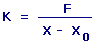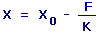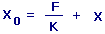Hooke's Law Calculators Enter value, select unit and click on calculate. Result will be displayed.

Calculate Force
Calculate Spring Constant
Calculate Distance from Equilibrium
Calculate Spring Equilibrium Position

 Web www.calculatoredge.com

Calculate Force

F = -K x (X - X0)

 F = Force K = Spring Constant X = Distance from Equilibrium X0 = Spring Equilibrium Position
 Enter your values: Spring Constant: Newton / Meter Pound / Foot Pound / Inch Distance from Equilibrium: Meter Centimeter Attometer Dekameter Decimeter Exameter Femtometer Foot Hectometer Inch Kilometer Megameter Micrometer Mile Millimeter Nanometer Petameter Picometer Yard Spring Equilibrium Position: Meter Centimeter Attometer Dekameter Decimeter Exameter Femtometer Foot Hectometer Inch Kilometer Megameter Micrometer Mile Millimeter Nanometer Petameter Picometer Yard Result: Force: Newton

Calculate Spring ConstantK = Spring Constant F = Force X = Distance from Equilibrium X0 = Spring Equilibrium Position
 Enter your values: Force: Newton Dyne Gram Force Kgs-Meter/sec^2 Kilograms Force Kip Pound Force Poundal Distance from Equilibrium: Meter Centimeter Attometer Dekameter Decimeter Exameter Femtometer Foot Hectometer Inch Kilometer Megameter Micrometer Mile Millimeter Nanometer Petameter Picometer Yard Spring Equilibrium Position: Meter Centimeter Attometer Dekameter Decimeter Exameter Femtometer Foot Hectometer Inch Kilometer Megameter Micrometer Mile Millimeter Nanometer Petameter Picometer Yard Result: Spring Constant: Newton/Meter

Calculate Distance from EquilibriumX = Distance from Equilibrium X0 = Spring Equilibrium Position K = Spring Constant F = Force
 Enter your values: Spring Equilibrium Position: Meter Centimeter Attometer Dekameter Decimeter Exameter Femtometer Foot Hectometer Inch Kilometer Megameter Micrometer Mile Millimeter Nanometer Petameter Picometer Yard Force: Newton Dyne Gram Force Kgs-Meter/sec^2 Kilograms Force Kip Pound Force Poundal Spring Constant: Newton / Meter Pound / Foot Pound / Inch Result: Distance from Equilibrium: Meter

Calculate Spring Equilibrium PositionX0 = Spring Equilibrium Position X = Distance from Equilibrium K = Spring Constant F = Force
 Enter your values: Force: Newton Dyne Gram Force Kgs-Meter/sec^2 Kilograms Force Kip Pound Force Poundal Spring Constant: Newton / Meter Pound / Foot Pound / Inch Distance from Equilibrium: Meter Centimeter Attometer Dekameter Decimeter Exameter Femtometer Foot Hectometer Inch Kilometer Megameter Micrometer Mile Millimeter Nanometer Petameter Picometer Yard Result: Spring Equilibrium Position: MeterLINKSDISCLAIMERCONTACT US